Math - 2014 Past Year Paper

# Math - 2014 Past Year Paper

Test Description

## 35 Questions MCQ Test IIT JAM Past Year Papers and Model Test Paper (All Branches) | Math - 2014 Past Year Paper

Math - 2014 Past Year Paper for IIT JAM 2022 is part of IIT JAM Past Year Papers and Model Test Paper (All Branches) preparation. The Math - 2014 Past Year Paper questions and answers have been prepared according to the IIT JAM exam syllabus.The Math - 2014 Past Year Paper MCQs are made for IIT JAM 2022 Exam. Find important definitions, questions, notes, meanings, examples, exercises, MCQs and online tests for Math - 2014 Past Year Paper below.
Solutions of Math - 2014 Past Year Paper questions in English are available as part of our IIT JAM Past Year Papers and Model Test Paper (All Branches) for IIT JAM & Math - 2014 Past Year Paper solutions in Hindi for IIT JAM Past Year Papers and Model Test Paper (All Branches) course. Download more important topics, notes, lectures and mock test series for IIT JAM Exam by signing up for free. Attempt Math - 2014 Past Year Paper | 35 questions in 90 minutes | Mock test for IIT JAM preparation | Free important questions MCQ to study IIT JAM Past Year Papers and Model Test Paper (All Branches) for IIT JAM Exam | Download free PDF with solutions
 1 Crore+ students have signed up on EduRev. Have you?
Math - 2014 Past Year Paper - Question 1

### Let f(x) = |x2 – 25| for all x ∈ R. The total number of points of R at which f attains a local extremum (minimum or maximum) is

Math - 2014 Past Year Paper - Question 2

### The coefficient of (x – 1)2 in the Taylor series expansion of f(x) = xex (x ∈ R) about the point x = 1 is

Math - 2014 Past Year Paper - Question 3

###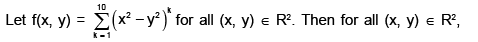Math - 2014 Past Year Paper - Question 4

For a, b, c ∈ R, if the differential equation (ax2 + bxy + y2)dx + (2x2 + cxy + y2)dy = 0 is exact, then

Math - 2014 Past Year Paper - Question 5

If f(x, y, z) = x2y + y2z + z2x for all (x, y, z) ∈ R3 and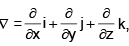then the value of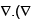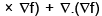at (1, 1, 1) is

Math - 2014 Past Year Paper - Question 6

The value of the radius of convergence of f(n) = 2n, n<0 is ____________

Detailed Solution for Math - 2014 Past Year Paper - Question 6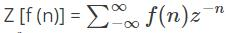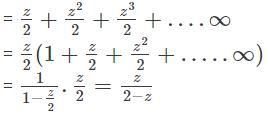The series is convergent if | z / 2 | < 1, that is | z | < 2

Hence, ROC is | z | < 2.

Math - 2014 Past Year Paper - Question 7

Let G be a group of order 17. The total number of non- isomorphic subgroups of G is

Detailed Solution for Math - 2014 Past Year Paper - Question 7

As 17 is a prime, and the order of a subgroup must divide the order of the group, G has only two subgroups, the unity subgroup and G itself. So the answer is 2.

Math - 2014 Past Year Paper - Question 8

Which one of the following is a subspace of the vector space R3 ?

Math - 2014 Past Year Paper - Question 9

Let T : R3 →  R3 be the linear transformation defined by T(x, y, z) = (x + y, z + z, z + x) for all (x, y, z) ∈ R3. Then

Math - 2014 Past Year Paper - Question 10

Let f:  R → R be a continuous function satisfying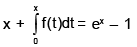for all x ∈ R . Then the set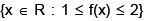is the interval

Math - 2014 Past Year Paper - Question 11

Directions: Q.11 – Q.35 carry two marks each.

The system of linear equations

x – y + 2z = b1

x + 2y – z = b2

2y – 2z = b3
is inconsistent when (b1, b2, b3) equals

Math - 2014 Past Year Paper - Question 12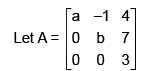be a matrix with real entries. If the sum and the product of all the eigenvalues
of A are 10 30 respectively, then a2 + b2 equals

Math - 2014 Past Year Paper - Question 13

Consider the subspace W = {(x1, x2, ..., x10) ∈ R10 : x n = xn–1 + xn–2 for 3 ≤  n ≤ 10} of the vector space R10. The dimension of W is

Math - 2014 Past Year Paper - Question 14

Let y1(x) and y2(x) be two linearly independent solutions of the differential equation

x2 y”(x) – 2xy’(x) – 4y(x) = 0 for x ∈ [1, 10].

Consider the Wronskian W(x) = y1(x)y2’(x) – y2(x)y1’(x). If W(1) = 1, then W(3) – W(2) equals

Math - 2014 Past Year Paper - Question 15

The equation of the curve passing through the point ,(π/2, 1) and having slope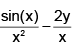at each
point (x, y) with x ≠ 0 is

Math - 2014 Past Year Paper - Question 16

The value of α ∈ R for which the curves x2 + αy2 = 1 and y = x2 intersect orthogonally is

Math - 2014 Past Year Paper - Question 17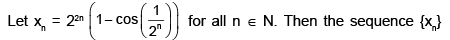Math - 2014 Past Year Paper - Question 18

Let {xn} be a sequence of real numbers such that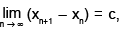where c is a positive real number. Then then sequence {xn /n} is

Math - 2014 Past Year Paper - Question 19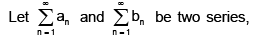where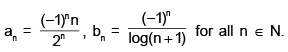Then

Math - 2014 Past Year Paper - Question 20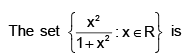Math - 2014 Past Year Paper - Question 21

The set all limit points of the set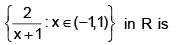Math - 2014 Past Year Paper - Question 22

Let S = [0, 1] ∪  [2, 3) and let f : S → R be defined by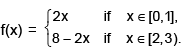If T = {f(x) : x ∈ S}, then the inverse function f–1 : T → S

Math - 2014 Past Year Paper - Question 23

Let f(x) = x3 + x and g(x) = x3 – x for all x ∈ R. If f–1 denotes the inverse function of f, then the derivative of the composite function g o f–1 at the point 2 is

Math - 2014 Past Year Paper - Question 24

For all (x, y) ∈ R2 ,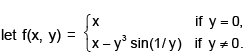Then at the point (0, 0),

Math - 2014 Past Year Paper - Question 25

For all (x, y)  ∈ R2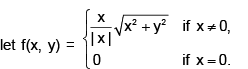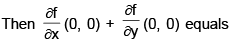Math - 2014 Past Year Paper - Question 26

Let f : R →  R be a function with continuous derivative such that f ( √2 ) = 2 and f(x) =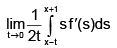for all x ∈ R. Then f(3) equals

Math - 2014 Past Year Paper - Question 27

The value of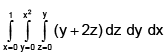is

Math - 2014 Past Year Paper - Question 28

If C is a smooth curve in R3 from (–1, 0, 1) to (1, 1, –1), then the value of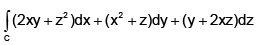is

Math - 2014 Past Year Paper - Question 29

Let C be the boundary of the region R = {(x, y) ∈ R2 : –1 ≤ y ≤ 1, 0 ≤ x ≤ 1 – y2} oriented in the counterclockwise direction. Then the value of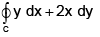Math - 2014 Past Year Paper - Question 30

Let G be a cyclic group of order 24. The total number of group isomorphism’s of G onto itself is

Math - 2014 Past Year Paper - Question 31

Let Sn be the group of all permutations on the set {1, 2, ..., n} under the composition of mappings.
For n > 2, if H is the smallest subgroup of Sn containing the transposition (1, 2) and the cycle (1, 2, ..., n), then

Math - 2014 Past Year Paper - Question 32

Let S be the oriented surface x2 + y2 + z2 = 1 with the unit normal n pointing outward. For the vector field F(x, y, z) = xi + yj + zk, the value of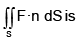Math - 2014 Past Year Paper - Question 33

Let f : (0, ∞ ) → R be a differentiable function such that f'(x2) = 1 - x3 for all x > 0 and f(1) = 0.
Then f(4) equals

Math - 2014 Past Year Paper - Question 34

Which one of the following conditions on a group G implies that G is abelian?

Math - 2014 Past Year Paper - Question 35

Let S = {x ∈ R : x6 – x5 ≤ 100} and T = {x2 – 2x : x ∈ (0, ∞)}. Then set S ∩ T is

## IIT JAM Past Year Papers and Model Test Paper (All Branches)

48 tests
 Use Code STAYHOME200 and get INR 200 additional OFF Use Coupon Code
Information about Math - 2014 Past Year Paper Page
In this test you can find the Exam questions for Math - 2014 Past Year Paper solved & explained in the simplest way possible. Besides giving Questions and answers for Math - 2014 Past Year Paper, EduRev gives you an ample number of Online tests for practice

48 tests